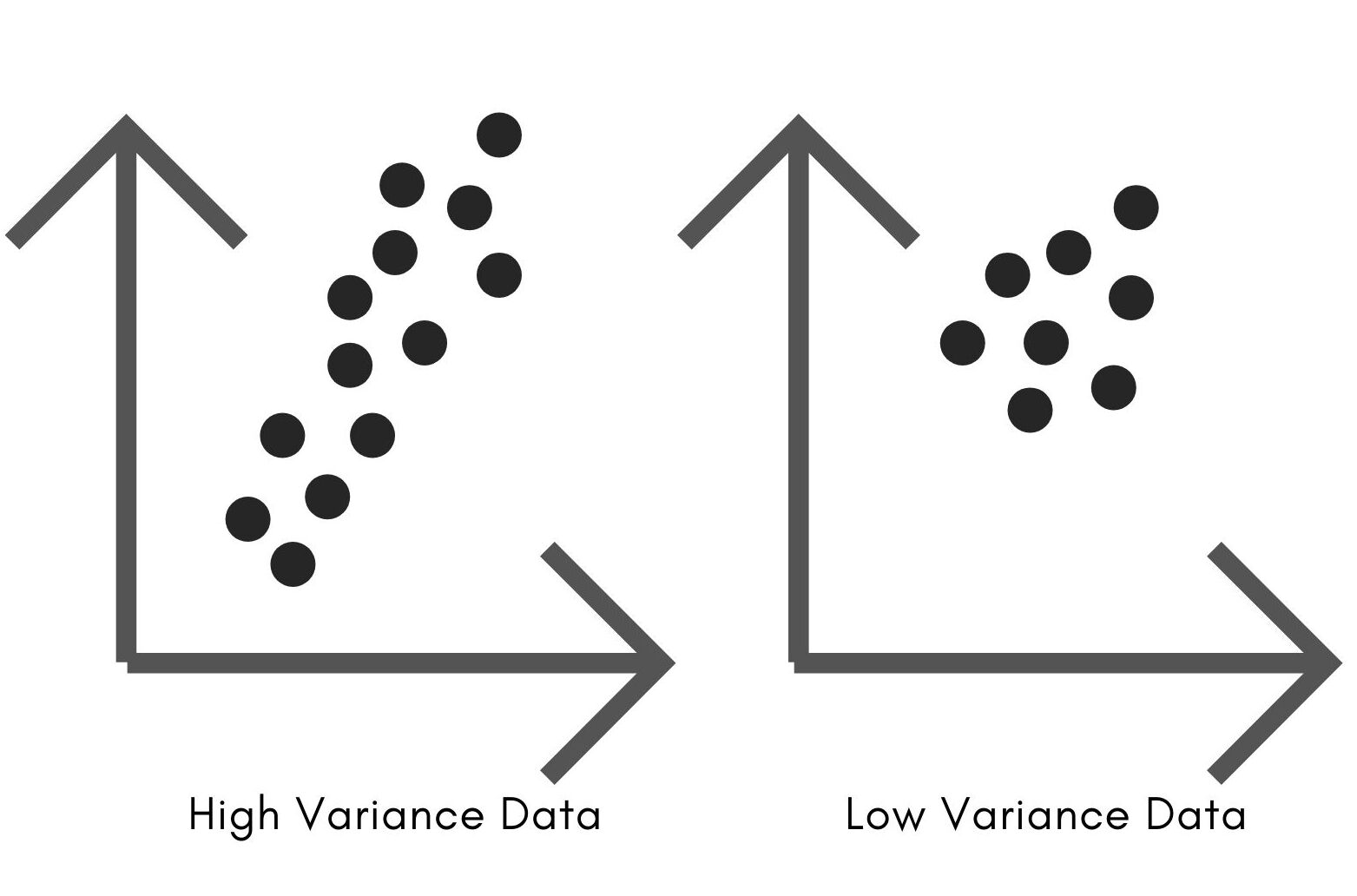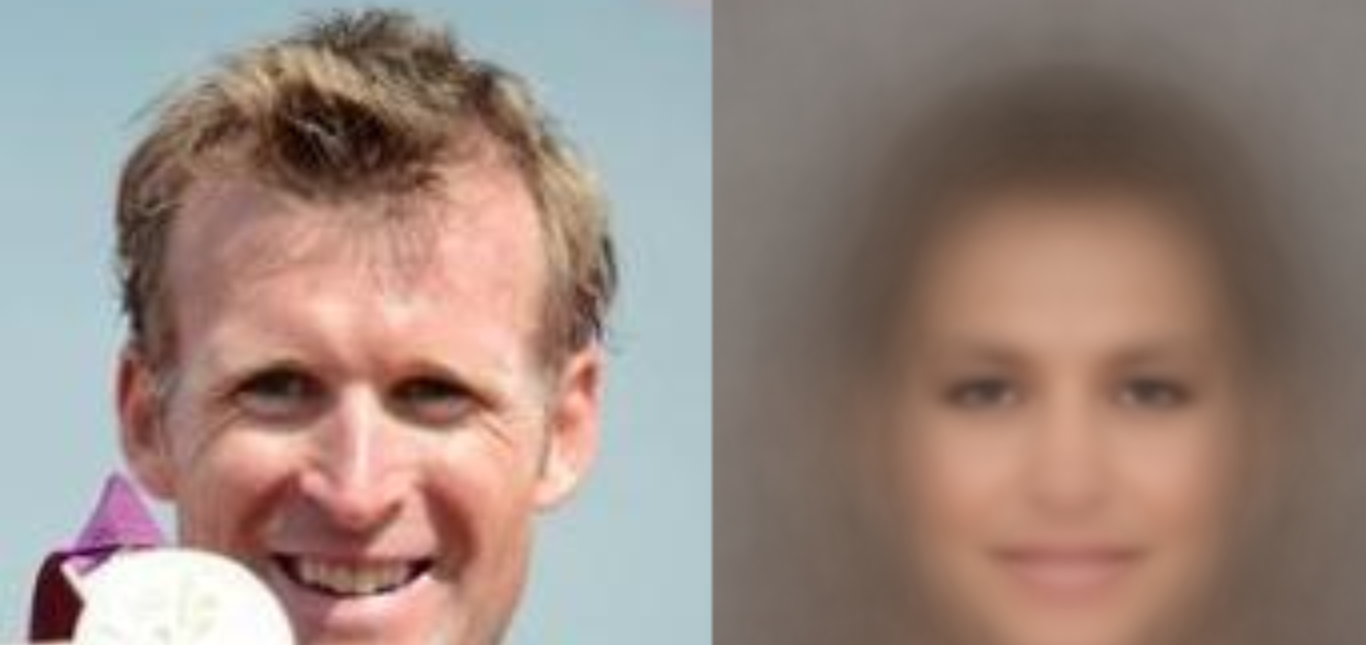×

Search anything:

# Find set of faces when combined results in face of person A

#### Machine Learning (ML) principal component analysisGet this book -> Problems on Array: For Interviews and Competitive Programming

In this article, we have demonstrated a mini-project where we Find a set of faces when combined results in face of person A. We do this using Machine Learning techniques and will give you a good idea of applications of ML.

1. Principal Component Analysis
2. Reconstructing the face using the EigenFaces
3. Training the Model
4. Testing the Model

Prerequisite: Principal Component Analysis

For accomplishing this project, we would start by understanding the Principal Component Analysis since it plays the vital role in developing the algorithm.

## Principal Component Analysis

It is one of the most important and popular dimentionality reduction technique in Machine Learning. In multi deimentional data, PCA allows us tro find dimensions that contain vital information for our model training.

Variance is the building block of PCA. Variance encodes the inoformation that is contained in the data. For a given n coordinates (a,b), we would need 2n numbers to represent such data. The axis which has more variance along with it has more data stored along with it. If there is no variance along with one axis then a single number can represent all information in n points along that axis.Our aim is to get all information of the data in few dimensions. In a two dimentional plane, there can be infinite number of directions to choose the Principal Component from and the second Principal Component can be chosen from the direction that has maximum variance of the plane. The third component could be chosen from the direction perpendicularto first and second pricipal components.
We could optionally drop some coordinates that have less information since we would want to retain the maximum information containing coordinates to represent data.

### Calculating the PCA

The principal components of given data could be found using the linear algebra. We can refer to PCA class in OpenCV to find the Principal Component Analysis. Multiple ways could be used to find the PCA :

• Assemble the Matrix of Data : We can start by assembling the data points into a matrix. One data point could be represented by one column.
• Mean Value Calculation : We can then calculate the mean value of all the data values. Depending upon the number of dimensions in the data, the mean will contain same number of dimensions.
• Subtracting mean from the Matrix of Data : We can subtract the mean value from all the data points, creating a fresh matrix.
• Covariance Calculation : Covariance gets the information about the spread of data,since we want the direction of maximum variance. The principal components are given by the eigen vectors of the matrix of covariance.

# Reconstructing the face using the EigenFaces

EigenFaces : They are calculated by estimating the principal components of the dataset containing the facial images. In our project, we would use the samples from the CalebA Dataset to train our model. For any image of 100 x 100 dimensions, it would have 100 x 100 x 3 numbers.
Hence, making a one dimensional array of thirty thousand elements.

To calculate the eigen faces we would be required to perform several steps:

• Get the Dataset containing the facial images
• Resize and adjust those images
• Create the matrix of data
• Perform the PCA to calculate weights:
• Start by vectorizing the images
• Then we subtract the mean vectors
• Then we calculate the dot product of mean of vectors that have been subtracted with each principal component.
• Multiply each weight to the Eigen Faces
• Resize and align the facial images
• Get the Eigen Faces via Eigen Vectors

## Training the Model

We start to train our model by using two functions :

1. readImage(path) : This function would read the sample images from our "samples" folder which contains 210 images from the CalebA Dataset. We create an array of our images. We would list all points in the directory. Then we read all points from the text files. Then we add them to our array of images that we created. We convert our images to float values and add them to the list. Then we would flip the images and append them. If no images are found in the mentioned folder then an error message is returned.
2. matrix(images) : This function would create the data matrix for our sample images. Since each image has three colour channels : Red, Green and Blue, hence the space taken by an image would be equivalent to its height x width x 3.

We start our python file by creating a directory referring to the folder containing the sample images. Then we would read those images and their size. Then we call the matrix function to accomplish our PCA calculations and the calculate the eigen vectors.

## Testing the Model

We use two functions to train our model :
1.reconstruction() : Using this function we could reconstruct our face of person A using the mean faces and Eigen Faces. As mentioned above we start by calculating the weights. The weights are the dot product of the mean image vactors that are subtracted with Eigen Vectors.
2. disp() : This function is used to display the original image against the reconstructed image as a result in new window.

We start the program by reading the model created pca_parameters.yml. The we calculate the mean vectors, eigen vectors and size of all images that we used during the training.

Then we calculate the mean vector and use it for our average face calculations. Then we get our eigen faces. For the purpose of testing we store an image in our test folder that was not used during the training of the data. We store the location in the variable image_file. Then we convert the test image into a vector and subtract mean vector. We create a new window to show the results.

The below image represents the final results that we have got :Hence by using above techniques in this article at OpenGenus, we can easily reconstruct the face of person A from set of faces.#### Srishti Guleria

Srishti Guleria has been a Technical Author, Software Developer, Intern at OpenGenus and a Computer Science student at Rajasthan Technical University, Kota